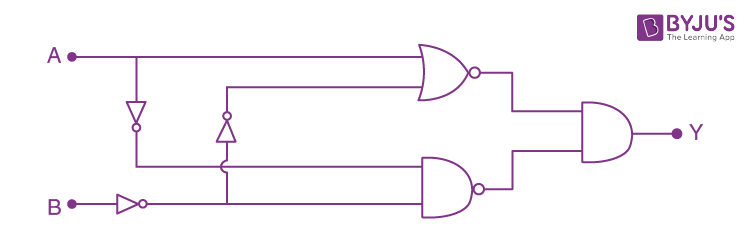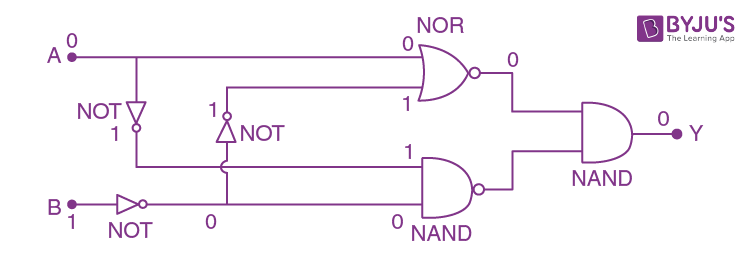Win up to 100% scholarship on Aakash BYJU'S JEE/NEET courses with ABNAT Win up to 100% scholarship on Aakash BYJU'S JEE/NEET courses with ABNAT

# JEE Main 2021 March 16 – Shift 1 Physics Question Paper with Solutions

JEE Main 2021 Physics question paper for March 16, shift 1 exam is provided here. Candidates will find solutions for each of the questions and these will help them to check the correct answers as well as analyze their performance. Students can either view all the question covered in the March 16 JEE Main 2021 Physics shift 1 question paper directly on the webpage or they can download it in the form of PDF for offline use. All the solutions have been prepared by our subject experts to help students grasp the concepts more clearly and also develop adept problem-solving skills for the entrance exam.

JEE Main 2021 March 16th Shift 1 Physics Question Paper

Section – A

Question 1. A 25 m long antenna is mounted on an antenna tower. The height of the antenna tower is 75 m. The wavelength (in meter) of the signal transmitted by this antenna would be:

a. 200
b. 400
c. 100
d. 300

Given that, the height of the peak of the antenna: H = 25 m.

As we know that

λ = 4H

Therefore, λ = 4×25

Therefore, λ = 100 m

Hence, option (c) is correct.

Question 2. A block of mass m slides along a floor while a force of magnitude F is applied to it at an angle θ as shown in the figure. The coefficient of kinetic friction is μK. Then, the block’s acceleration ‘a’ is given by (g is the acceleration due to gravity)a.

$$\begin{array}{l}\frac{F}{m}cos\theta -\mu _{k}(g-\frac{F}{m}sin\theta )\end{array}$$

b.
$$\begin{array}{l}\frac{F}{m}cos\theta -\mu _{k}(g+\frac{F}{m}sin\theta )\end{array}$$

c.
$$\begin{array}{l}\frac{F}{m}cos\theta +\mu _{k}(g-\frac{F}{m}sin\theta )\end{array}$$

d.
$$\begin{array}{l}-\frac{F}{m}cos\theta -\mu _{k}(g-\frac{F}{m}sin\theta )\end{array}$$

Drawing the FBD of the block.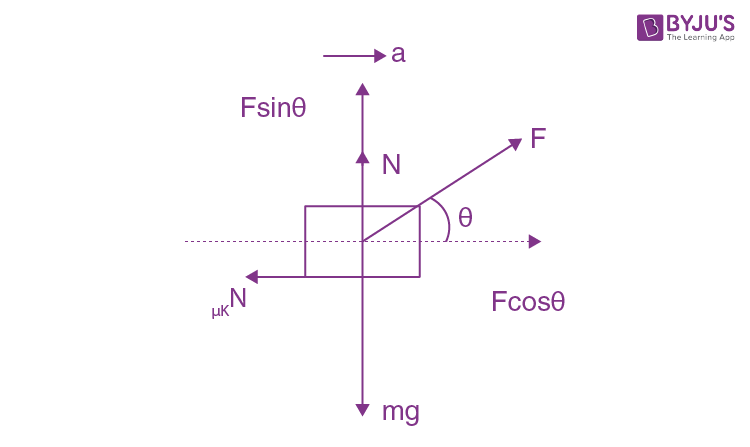⇒ N = mg – Fsinθ …(1)

Also, Fcosθ – μKN = m⋅a …(2)

Substituting the value of N from eq. (1) in eq. (2)

⇒ Fcosθ – μK(mg–Fsinθ) = m⋅a

⇒ a =(F/m)cosθ- μK(g- (F/m)sinθ)

Hence, option (a) is correct.

Question 3. Four equal masses, m each is placed at the corners of a square of length(l) as shown in the figure. The moment of inertia of the system about an axis passing through A and parallel to DB would be: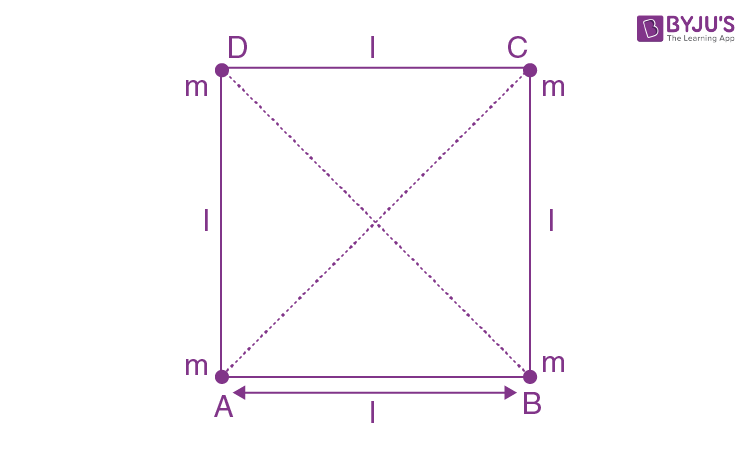a.ml2
b.3ml2
c.√3ml2
d.2ml2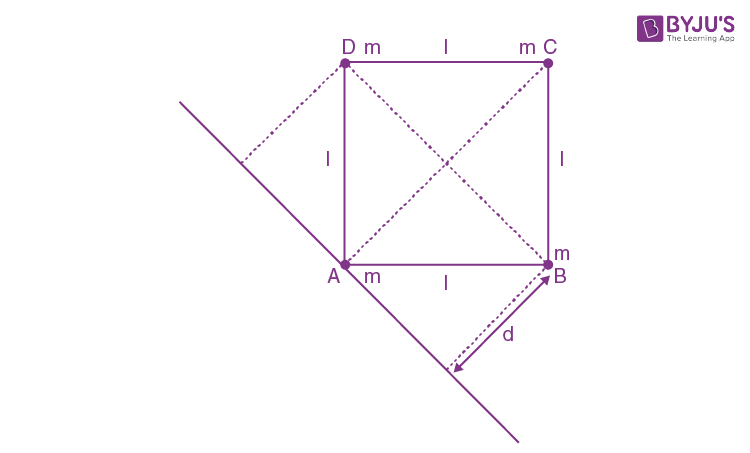$$\begin{array}{l}AC= \sqrt{l^{2}+l^{2}}\end{array}$$

AC = l√2

d =( l√2)/2

⇒ l/√2

Moment of inertia about the axis passing through A :

I = m(0)2 + m(d)2 + m(d)2 + m(AC)2

⇒ I = 0 + m(l/√2)2 + m(l/√2)2+ m(l√2)2

⇒I = ml2/2 + ml2/2 + 2ml2

⇒ I = 3ml2

Hence, option (b) is correct.

Question 4. The stopping potential in the context of the photoelectric effect depends on the following property of incident electromagnetic radiation:

a. Amplitude
b. Phase
c. Frequency
d. Intensity

According to Einstein’s photoelectric equation, stopping potential depends on frequency as

h

$$\begin{array}{l}\nu\end{array}$$
– h
$$\begin{array}{l}\nu\end{array}$$
0 = eV

⇒V= (h/e)

$$\begin{array}{l}\nu\end{array}$$
-(h/e)
$$\begin{array}{l}\nu\end{array}$$
0

Hence stopping potential depends on frequency.

Hence, option (c) is correct.

Question 5. One main scale division of a vernier callipers is ‘a’ cm and nth division of the vernier scale coincides with (n–1)th division of the main scale. The least count of the callipers in mm is:

a.

$$\begin{array}{l}\left ( \frac{n-1}{10n} \right )a\end{array}$$

b.10a/n
c.10na/(n-1)
d. 10a/(n-1)

MSD → Main scale division

VSD → Vernier scale division

LC → Least count

n VSD = (n–1) MSD

1  VSD= [(n-1)/n] MSD

L⋅C=1 MSD – 1 VSD

=1 MSD- [(n-1)/n]MSD

=1 MSD-1 MSD+ MSD/n

= MSD/n

=(a/n)cm

=(10a/n) mm

Hence, option (b) is correct.

Question 6. A plane electromagnetic wave of frequency 500 MHz is travelling in vacuum along y-direction. At a particular point in space and time,

$$\begin{array}{l}\vec{B}=8.0\times 10^{-8}\hat{z}T\end{array}$$
. The value of electric field at this point is: (speed of light = 3×108 ms–1) Assume x, y, z are unit vectors along
$$\begin{array}{l}\hat{x}\end{array}$$
,
$$\begin{array}{l}\hat{y}\end{array}$$
and
$$\begin{array}{l}\hat{z}\end{array}$$
directions.

a.

$$\begin{array}{l}2.6\hat{x}\frac{V}{m}\end{array}$$

b.
$$\begin{array}{l}-2.6\hat{y}\frac{V}{m}\end{array}$$

c.
$$\begin{array}{l}24\hat{x}\frac{V}{m}\end{array}$$

d.
$$\begin{array}{l}-24\hat{x}\frac{V}{m}\end{array}$$

E0 = B⋅C

E0 = (8×10–8) × (3×108)

⇒ |E0| = 24

Wave travels in the direction of

$$\begin{array}{l}\vec{E}\times \vec{B}\end{array}$$

As

$$\begin{array}{l}(-\hat{x})\times \hat{z}=+\hat{y}\end{array}$$

Therefore,

$$\begin{array}{l}\hat{E}=-24\hat{x}V/m\end{array}$$

Hence, option (d) is correct.

Question 7. The maximum and minimum distances of a comet from the Sun are 1.6×1012 m and 8.0×1010 m respectively. If the speed of the comet at the nearest point is 6×104 ms–1, the speed at the farthest point is :

a. 1.5×103 m/s
b. 4.5×103 m/s
c. 3.0×103 m/s
d. 6.0×103 m/s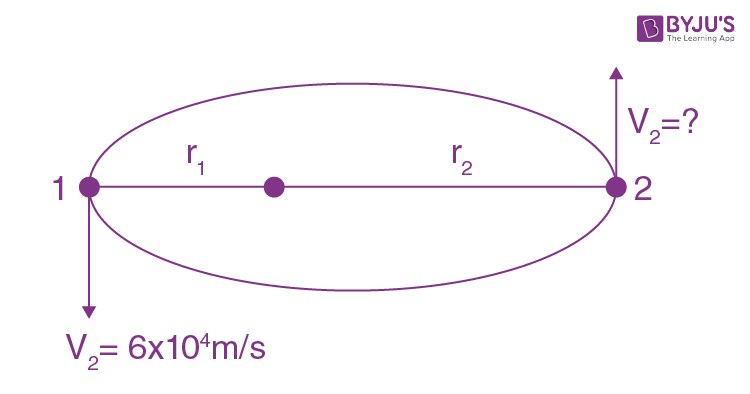Let point 1 is nearest point,

and point 2 is the farthest point.

Given, r1 = 8×1010 m & r2 = 1.6×1012 m

By angular momentum conservation

L1 = L2

mr1v1= mr2v2

$$\begin{array}{l}v_{2}=\frac{r_{1}v_{1}}{r_{2}}\end{array}$$
$$\begin{array}{l}v_{2}=\frac{8\times 10^{10}\times 6\times 10^{4}}{1.6\times 10^{12}}\end{array}$$

∴v2 = 3.0×103 m/s

Hence, option (c) is correct.

Question 8. A block of 200 g mass moves with a uniform speed in a horizontal circular groove, with vertical sidewalls of a radius of 20 cm. If the block takes 40 s to complete one round, the normal force by the sidewalls of the groove is:

a. 6.28 ×10–3 N
b. 0.0314 N
c. 9.859×10–2 N
d. 9.859×10–4 N

Normal force will provide the necessary centripetal force.

⇒ N=mω2R

Also; ω=2π/T

$$\begin{array}{l}N = (0.2)\left ( \frac{4\pi ^{2}}{T^{2}}(0.2) \right )\end{array}$$
$$\begin{array}{l}=(0.2)(\frac{4\times 3.14 ^{2}}{(40)^{2}}(0.2)\end{array}$$

Therefore, N=9.859×10–4 N

Hence, option (d) is correct.

Question 9. An RC circuit as shown in the figure is driven by an AC source generating a square wave. The output wave pattern monitored by CRO would look close to: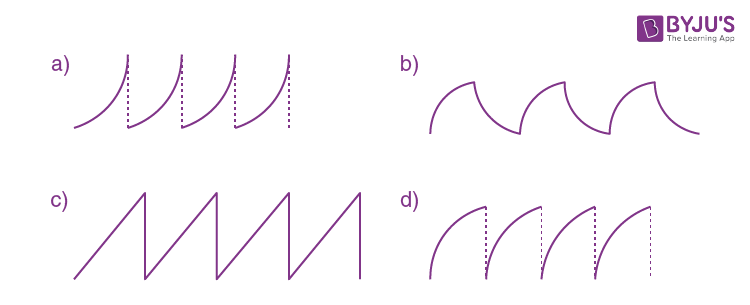Assuming AC starts with positive voltage. When +ve voltage is across input then the capacitor starts charging, trying to reach saturation value, till then there is +ve voltage across the input. When –ve voltage of AC appears across the input, the capacitor starts discharging till then there is –ve voltage across the input and this process of charging and discharging keeps on going alternatively.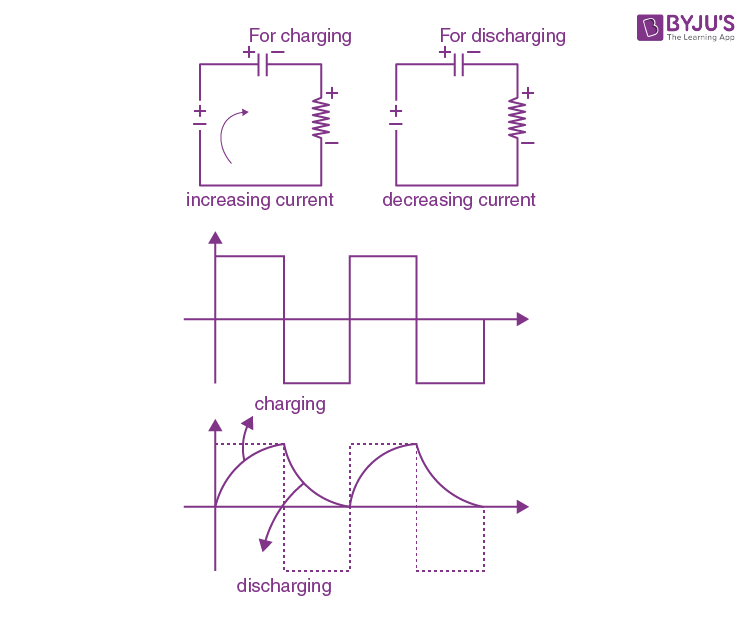Hence, option (b) is correct.

Question 10. In thermodynamics, heat and work are:

a. Intensive thermodynamics state variables
b. Extensive thermodynamics state variables
c. Path functions
d. Point functions

Heat and work are path functions. Heat and work depend on the path taken to reach the final state from the initial state. Intensive and extensive properties only apply to physical properties that are a function of the state, heat is neither intensive nor extensive. Hence option (c) is correct.

Question 11. A conducting wire of length ‘l’, area of cross-section A and electric resistivity ρ is connected between the terminals of a battery. A potential difference V is developed between its ends, causing an electric current. If the length of the wire of the same material is doubled and the area of cross-section is halved, the resultant current would be:

a.

$$\begin{array}{l}\frac{1}{4}\frac{\rho l}{VA}\end{array}$$

b.
$$\begin{array}{l}\frac{3}{4}\frac{VA}{\rho l}\end{array}$$

c.
$$\begin{array}{l}4\frac{VA}{\rho l}\end{array}$$

d.
$$\begin{array}{l}\frac{1}{4}\frac{VA}{\rho l}\end{array}$$

We know that

R=ρ(l/A)

Now, new length: l’=2l

New area of cross-section: A’=A/2

Therefore, New resistance :

$$\begin{array}{l}R’ = \rho \frac{2l}{\frac{A}{2}}\end{array}$$

⇒R’=4(ρl/A)

⇒ R’=4R

Therefore, Resultant current: I=V/4R

I= (1/4)(VA/ρl)

Hence, option (d) is correct.

Question 12. The pressure acting on a submarine is 3×105 Pa at a certain depth. If the depth is doubled, the percentage increase in the pressure acting on the submarine would be :(Assume that atmospheric pressure is 1×105 Pa, the density of water is 103 kg m–3, acceleration due to gravity g = 10 ms–2)

a. (200/3)%
b. (5/200)%
c. (200/5)%
d. (3/200)%

Pressure at depth h is

P = P0 +hρg= 3×105 Pa

⇒ hρg = 3×105–1×105

⇒ hρg = 2×105

As h is doubled

∴ 2hρg = 4×105

∴ Increased pressure, P’=P0+4×105

∴ P’=5×105Pa

∴% increase in pressure =(P’-P/P)×100

$$\begin{array}{l}= \frac{(5-3)\times 10^{5}}{3\times 10^{5}}\times 100\end{array}$$

=(200/3) %

Hence option (a) is correct.

Question 13. A bar magnet of length 14 cm is placed in the magnetic meridian with its north pole pointing towards the geographic north pole. A neutral point is obtained at a distance of 18 cm from the centre of the magnet. If BH = 0.4 G, the magnetic moment of the magnet is (1 G = 10–4T)

a. 28.80 J T–1
b. 2.880 J T–1
c. 2.880×103 J T–1
d. 2.880×102 J T–1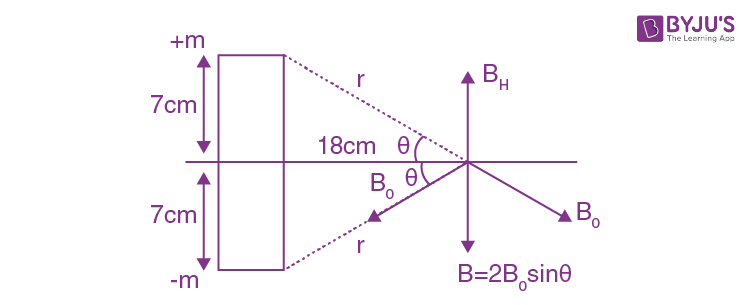M → magnetic moment of the magnet

m → power of magnetic pole

θ → angle made by B0 with the horizontal

B0 → magnetic flux density

B=2B0sinθ

$$\begin{array}{l}B=2\frac{\mu _{0}}{4\pi }\frac{m}{r^{2}}\times \frac{7}{r}\end{array}$$

$$\begin{array}{l}0.4\times 10^{-4}=2\times 10^{-7}\times \frac{m\times 7}{(7^{2}+18^{2})^{3/2}}\times 10^{4}\end{array}$$

Therefore,

$$\begin{array}{l}m= \frac{4\times 10^{-2}\times (373)^{3/2}}{14}\end{array}$$

M=m×14 cm=m×(14/100)

$$\begin{array}{l}M= \frac{4\times 10^{-2}\times (373)^{3/2}}{14}\times \frac{14}{100}\end{array}$$

M = 2.880 J/T

Hence option (b) is correct.

Question 14. The volume V of an enclosure contains a mixture of three gases, 16 g of oxygen, 28 g of nitrogen and 44 g of carbon dioxide at absolute temperature T. Consider R as a universal gas constant. The pressure of the mixture of gases is:

a.(4RT)/V
b.(88RT)/V
c.(5/2)(RT/V)
d. 3RTV

No. of moles of O2 : n1=16/32=0.5 mole

No. of moles of N2 : n2=28/28=1 mole

No. of moles of CO2: n3=44/44=1 mole

Total no. of moles in container : n=n1+n2+n3

∴n=0.5+1+1=(5/2)moles

Now; PV=nRT

P=(nRT)/V

P= (5/2)(RT/V)

Hence option (c) is correct.

Question 15. A conducting bar of length L is free to slide on two parallel conducting rails as shown in the figure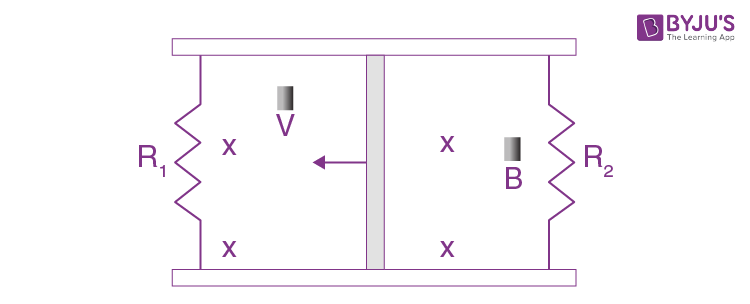Two resistors R1 and R2 are connected across the ends of the rails. There is a uniform magnetic field

$$\begin{array}{l}\vec{B}\end{array}$$
pointing into the page. An external agent pulls the bar to the left at a constant speed v. The correct statement about the directions of induced currents I1 and I2 flowing through R1 and R2 respectively is :

a. I1 is in the clockwise direction and I2 is in the anticlockwise direction
b. Both I1 and I2 are in a clockwise direction
c. I1 is in the anticlockwise direction and I2 is in a clockwise direction
d. Both I1 and I2 are in the anticlockwise direction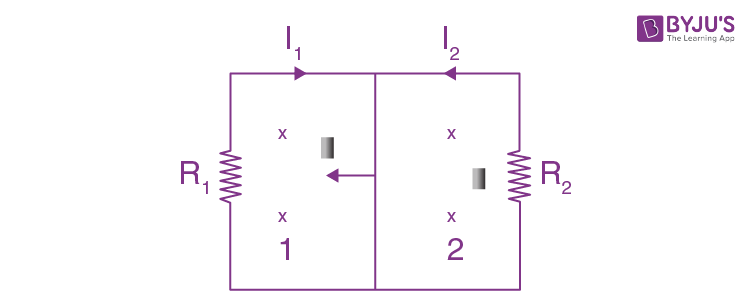When the bar slides, the area of loop 1 decreases and that of loop 2 increases. Magnetic flux decreases in 1 and increases in 2. Therefore induced emf and current resist this change. As a result, B should increase in 1 and decrease in 2. So I1 should be clockwise and I2 anticlockwise.

Hence option (a) is correct.

Question 16. The velocity-displacement graph describing the motion of a bicycle is shown in the figure.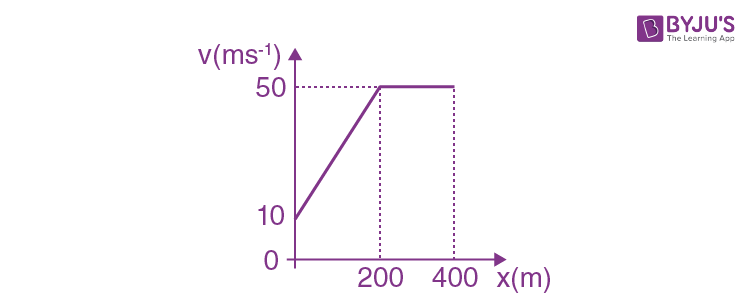The acceleration-displacement graph of the bicycle’s motion is best described by: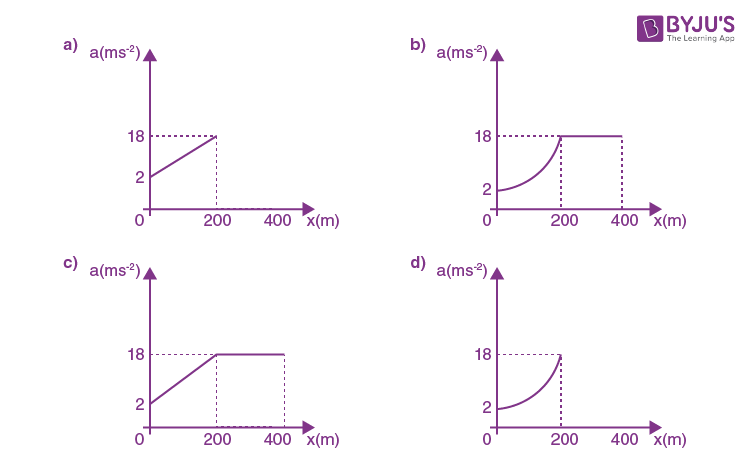We know that, a=v(dv/dx)

As slope is constant, so a ∝ v (from x=0 to 200 m) & Also, slope = 0, so a = 0 (from x=200 to 400 m)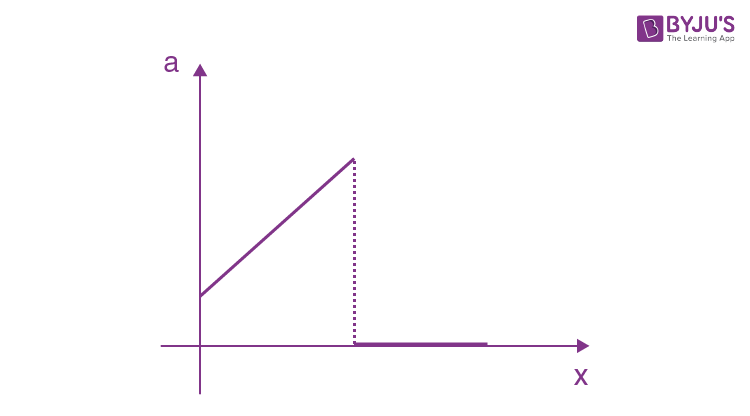Hence, the correct plot is a.

Question 17. For changing the capacitance of a given parallel plate capacitor, a dielectric material of dielectric constant K is used, which has the same area as the plates of the capacitor. The thickness of the dielectric slab is (¾) d, where ‘d’ is the separation between the plates of parallel plate capacitors. The new capacitance (C’) in terms of original capacitance (C0) is given by the following relation:

a.

$$\begin{array}{l}C’=\frac{4K}{K+3}C_{0}\end{array}$$

b.
$$\begin{array}{l}C’=\frac{4}{3+K}C_{0}\end{array}$$

c.
$$\begin{array}{l}C’=\frac{3+K}{4K}C_{0}\end{array}$$

d.
$$\begin{array}{l}C’=\frac{4+K}{3}C_{0}\end{array}$$$$\begin{array}{l}C_{0}=\frac{\epsilon _{0}A}{d}\end{array}$$

C1 and C2 are in series and C’ is new capacitance

Therefore,

$$\begin{array}{l}\frac{1}{C’} = \frac{1}{C_{1}}+\frac{1}{C_{2}}\end{array}$$
$$\begin{array}{l}\frac{1}{C’} = \frac{(3d/4)}{\epsilon _{0}KA}+\frac{d/4}{\epsilon _{0}A}\end{array}$$
$$\begin{array}{l}\frac{1}{C’} = \frac{d}{4\epsilon _{0}A}\left ( \frac{3+K}{K} \right )\end{array}$$

Therefore,

$$\begin{array}{l}C’= \frac{4K}{(K+3)}C_{0}\end{array}$$

Hence option (a) is correct.

Question 18. For an electromagnetic wave travelling in free space, the relation between average energy densities due to electric (Ue) and magnetic (Um) fields is :

a. Ue ≠ Um
b. Ue = Um
c. Ue > Um
d. Ue < Um

In EMW, the average energy density due to electric field (Ue) and magnetic field (Um) is the same. Hence option (b) is correct.

Question 19. Time period of a simple pendulum is T inside a lift when the lift is stationary. If the lift moves upwards with an acceleration g/2, the time period of the pendulum will be:

a.

$$\begin{array}{l}\sqrt{\frac{3}{2}}T\end{array}$$

b.
$$\begin{array}{l}\frac{T}{\sqrt{3}}\end{array}$$

c.
$$\begin{array}{l}\sqrt{\frac{2}{3}}T\end{array}$$

d.
$$\begin{array}{l}\sqrt{3}T\end{array}$$

When lift is stationary

$$\begin{array}{l}T=2\pi \sqrt{\frac{L}{g}}\end{array}$$

A pseudo force will act downwards when the lift is accelerating upwards.

Therefore,

$$\begin{array}{l}g_{eff}=g+\frac{g}{2}=\frac{3g}{2}\end{array}$$

New time period

$$\begin{array}{l}T’ = 2\pi \sqrt{\frac{L}{g_{eff}}}\end{array}$$
$$\begin{array}{l}T’ = 2\pi \sqrt{\frac{2L}{3g}}\end{array}$$

Therefore,

$$\begin{array}{l}T’ = \sqrt{\frac{2}{3}}T\end{array}$$

Hence option (c) is correct.

Question 20. The angle of deviation through a prism is minimum when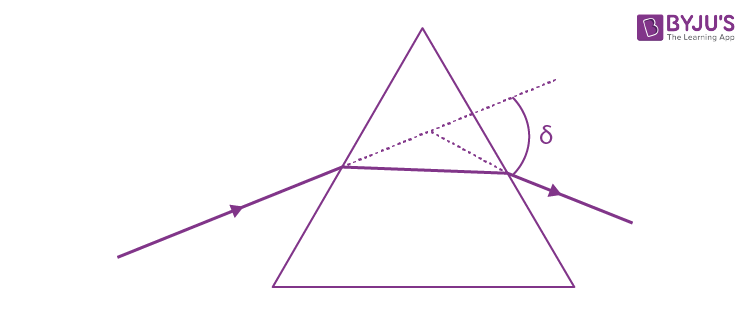(A) Incident ray and emergent ray are symmetric to the prism

(B) The refracted ray inside the prism becomes parallel to its base

(C) Angle of incidence is equal to that of the angle of emergence

(D) When the angle of emergence is double the angle of incidence

Choose the correct answer from the options given below:

a. Only statement (D) is true
b. Statements (A), (B) and (C) are true
c. Statements (B) and (C) are true
d. Only statement (A) and (B) are true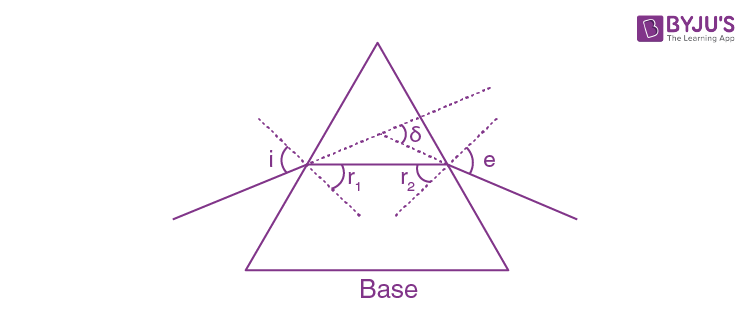Deviation is minimum in prism when, i = e, r1=r2 and ray inside prism is parallel to the base of the prism.

Hence option (b) is correct.

SECTION-B

Question 21. A fringe width of 6 mm was produced for two slits separated by 1 mm apart. The screen is placed 10 m away. The wavelength of light used is ‘x’ nm. The value of ‘x’ to the nearest integer is ________.

β = 6 mm, d =1 mm, D =10 m

λ = ?

$$\begin{array}{l}\beta =\frac{\lambda D}{d}\end{array}$$
$$\begin{array}{l}6\times 10^{-3} =\frac{\lambda \times 10}{1\times 10^{-3}}\end{array}$$
$$\begin{array}{l}\lambda =\frac{6\times 10^{-3}\times 1\times 10^{-3}}{10}\end{array}$$

λ = 600 × 10-9 m

∴ λ = 600 nm

∴ 600 is the required value.

Question 22. The value of power dissipated across the Zener diode (Vz = 15 V) connected in the circuit as shown in the figure is x*10–1 watt.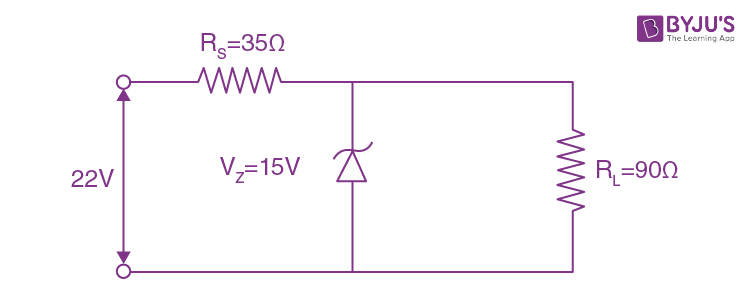The value of x, to the nearest integer, is _________.

Across R1 potential difference is 22 V – 15 V = 7 V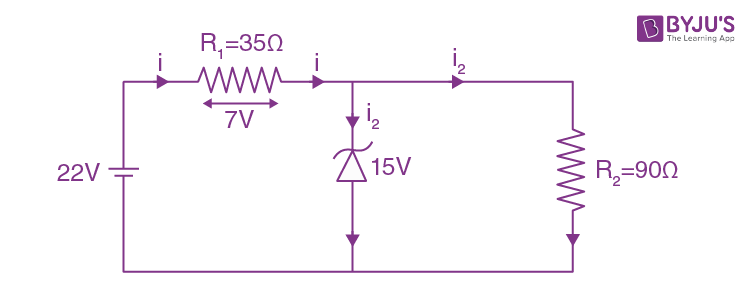$$\begin{array}{l}i=\frac{7}{35}=\frac{1}{5}A\end{array}$$
$$\begin{array}{l}i_{1}=\frac{15}{90}=\frac{1}{6}A\end{array}$$
$$\begin{array}{l}i_{2}=i-i_{1}\end{array}$$
$$\begin{array}{l}i_{2}=\frac{1}{5}-\frac{1}{6}\end{array}$$
$$\begin{array}{l}i_{2}=\frac{1}{30}A\end{array}$$

Power across diode ; P = V2 i2

P=15×(1/30)

P = 0.5 W

∴ P = 5×10–1 W

∴ 5 is the required value.

Question 23. The resistance R=V/I, where V = (50±2) V and I = (20±0.2) A. The percentage error in R is ‘x’ %. The value of ‘x’ to the nearest integer is _________.

R=V/I

(ΔR/R)×100=(ΔV/V) ×100+(ΔI/I)×100

% error in R=(2/50)×100+(0.2/20)×100

% error in R = 4+1

∴ % error in R = 5%

∴ 5 is the required value.

Question 24. A sinusoidal voltage of peak value 250 V is applied to a series LCR circuit, in which R = 8Ω, L=24 mH and C=60 μF. The value of power dissipated at resonant conditions is ‘x’ kW. The value of x to the nearest integer is _________.

At resonance, power (P)

P=(Vrms)2R

Therefore,

$$\begin{array}{l}P = \frac{\left ( \frac{250}{\sqrt{2}} \right )^{2}}{8}\end{array}$$

∴ P = 3906.25 W

∴ P ≅ 4 kW

∴ 4 is the required value.

Question 25. A ball of mass 10 kg moving with a velocity 10√3 ms–1 along the X-axis, hits another ball of mass 20 kg which is at rest. After the collision, the first ball comes to rest and the second one disintegrates into two equal pieces. One of the pieces starts moving along the Y-axis at a speed of 10 m/s. The second piece starts moving at a speed of 20 m/s at an angle θ (degree) with respect to the X-axis. The configuration of pieces after the collision is shown in the figure. The value of θ to the nearest integer is ________.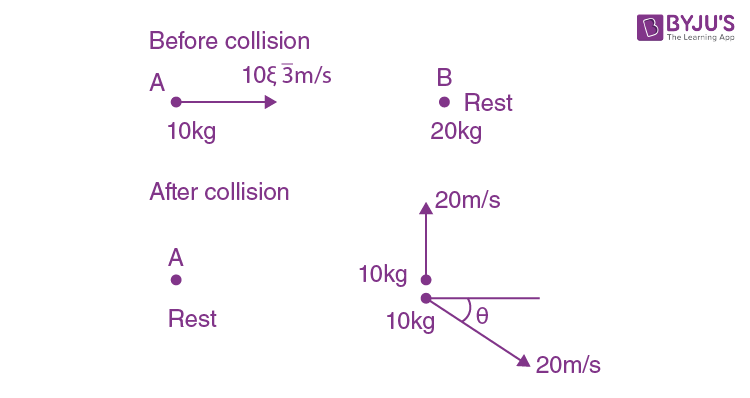Conserving momentum along x-axis

$$\begin{array}{l}\vec{p_{i}}=\vec{p_{f}}\end{array}$$
$$\begin{array}{l}10\times 10\sqrt{3}=10\times 20 cos\theta\end{array}$$
$$\begin{array}{l}cos\theta=\sqrt{3}/2\end{array}$$

Therefore,

θ = 30°

∴ 30 is the required value.

Question 26. In the figure given, the electric current flowing through the 5 kΩ resistor is ‘x’ mA.The value of x to the nearest integer is ________.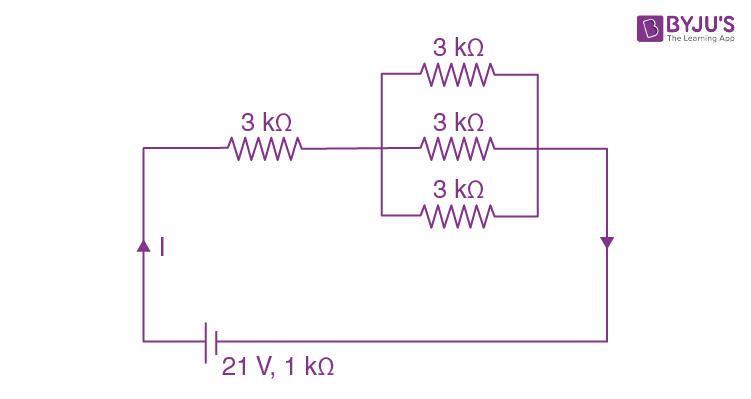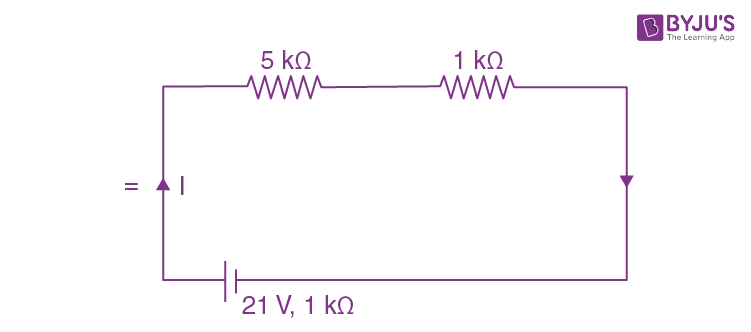I=21/(5+1+1)

∴ I = 3 mA

∴ 3 is the required value.

Question 27. Consider a 20 kg uniform circular disk of radius 0.2 m. It is pin supported at its centre and is at rest initially. The disk is acted upon by a constant force F=20 N through a massless string wrapped around its periphery as shown in the figure.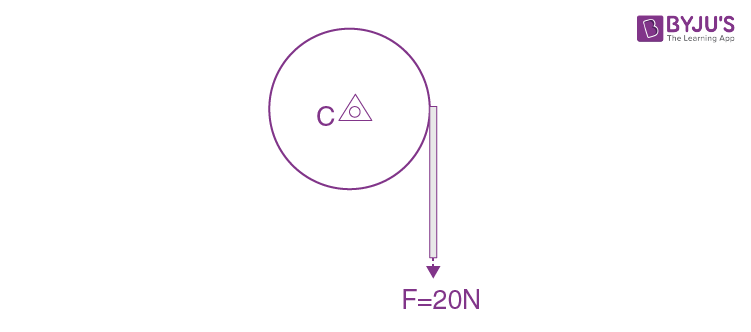Suppose the disk makes n number of revolutions to attain an angular speed of 50 rad s–1. The value of n, to the nearest integer is ________. [Given: In one complete revolution, the disk rotates by 6.28 rad]

$$\begin{array}{l}\alpha =\frac{\tau }{I}=\frac{FR}{mR^{2}/2}=\frac{2F}{mR}\end{array}$$
$$\begin{array}{l}\alpha =\frac{2\times 20}{20\times 0.2}\end{array}$$
$$\begin{array}{l}\omega ^{2}=\omega _{0}^{2}+2\alpha \triangle \theta\end{array}$$

(50)2 = 02 + 2(10) Δθ

⇒ Δθ = 2500/20

No. of revolution =125/2π≈20 revolutions.

∴ 20 is the required value.

Question 28. The first three spectral lines of H-atom in the Balmer series are given λ1, λ2, λ3 considering the Bohr atomic model, the wavelengths of first and third spectral lines (λ13) are related by a factor of approximately ‘x’×10–1. The value of x, to the nearest integer, is ________.

For 1st line

$$\begin{array}{l}\frac{1}{\lambda _{1}}=Rz^{2}(\frac{1}{2^{2}}-\frac{1}{3^{2}})\end{array}$$
$$\begin{array}{l}\frac{1}{\lambda _{1}}=Rz^{2}(\frac{5}{36})\end{array}$$
———(1)

For 3rd line

$$\begin{array}{l}\frac{1}{\lambda _{3}}=Rz^{2}(\frac{1}{2^{2}}-\frac{1}{5^{2}})\end{array}$$
$$\begin{array}{l}\frac{1}{\lambda _{3}}=Rz^{2}(\frac{21}{100})\end{array}$$
——-(2)

Therefore, dividing the latex 2 by 1

$$\begin{array}{l}\frac{\lambda _{1}}{\lambda _{3}}=\frac{21}{100}\times \frac{36}{5}= 1.512 = 15.12 \times 10^{-1}\end{array}$$

x ≈ 15

∴ 15 is the required value.

Question 29. Consider a frame that is made up of two thin massless rods AB and AC as shown in the figure. A vertical force

$$\begin{array}{l}\vec{P}\end{array}$$
of magnitude 100 N is applied at point A of the frame.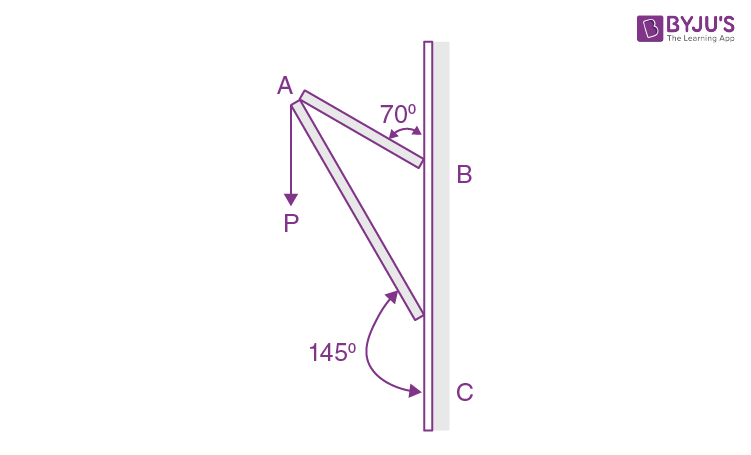Suppose the force is

$$\begin{array}{l}\vec{P}\end{array}$$
resolved parallel to the arms AB and AC of the frame. The magnitude of the resolved component along the arm AC is x N. The value of x, to the nearest integer, is ________. [Given: sin(35°)=0.573, cos(35°)=0.819, sin(110°)=0.939, cos(110°)=–0.342]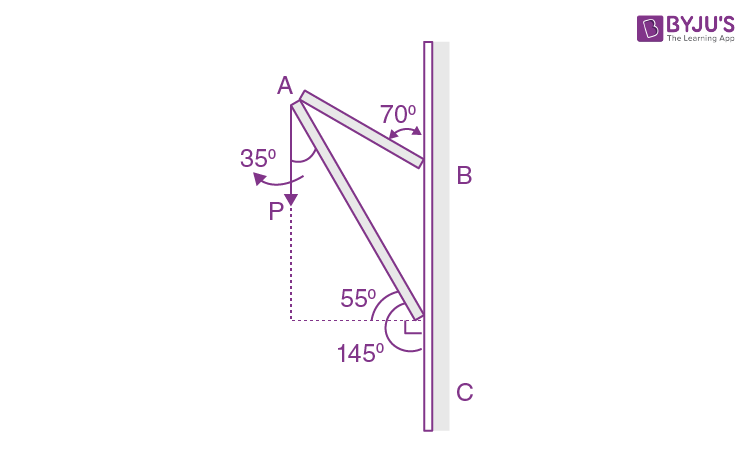Component along AC

= 100 cos 35°N

= 100 × 0.819 N

= 81.9 N

≈ 82 N

∴ 82 is the required value.

Question 30. In the logic circuit shown in the figure, if input A and B are 0 to 1 respectively, the output at Y would be ‘x’. The value of x is ________.